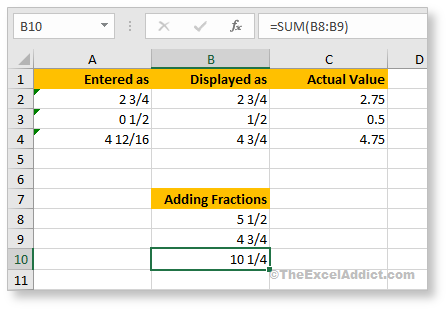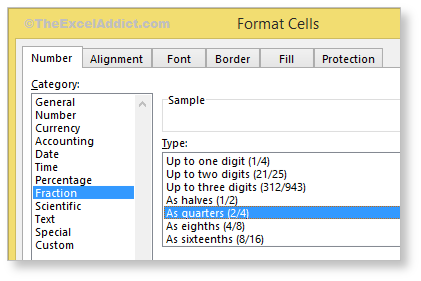# Using Fractions

Maybe you have tried using fractions in Excel and were left a little confused when dates started popping up.

The key to entering fractions in Excel is, you need to type a whole number, a space and then the fraction (e.g. 2 3/4).

For fraction values of less than 1 (e.g 1/2), you must enter a zero in place of the whole number, a space and then the fraction (e.g. 0 1/2). If you enter only the fraction part, Excel will likely interpret it as a date and display it as ‘02-Jan‘, unless you’ve already formatted your cell(s) to be displayed as fractions.To format cells with a fraction format, from the Home tab, select the number format dropdown in the Number group and choose Fraction.

Note that, even though the cell displays the number in a fraction format (e.g. 2 3/4), if you look in the Formula Bar you will see the decimal equivalent (e.g. 2.75). This enables you to do simple calculations with fractions even though the cells are formatted as fractions.

The cool thing about this is that when you enter a value that Excel interprets as a fraction, the fraction is automatically reduced to its smallest denominator (e.g. 4 12/16 will be displayed as 4 3/4).

To override this default format, open the Format Cells dialog (CTRL+1) and click Fraction. You will see several options available for formatting fractions.